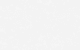# Modulus of Elasticity of Concrete Calculator

### Calculate the modulus of elasticity of concrete in accordance to different design codes.

Most design codes have different equations to compute the elastic modulus of concrete.

Ec = 0.00 MPa

## Brief Description

Modulus of elasticity is one of the most important properties of concrete, or any material for that matter, because it represents the capacity of the material to resist deformation under applied load. This property is the basis of our understanding of the strength of material and the foundation for all types of structural analysis.

Any structural engineer would be well-versed of the definition and use of modulus of elasticity (sometimes called Young’s Modulus). You may be familiar with the stress-strain diagram below. The linear portion of the curve represents the elastic region of deformation by which the modulus of elasticity, Ec is expressed as the ratio of stress against strain. This is just one of several model curves adopted by codes.

This online calculator allows you to compute the modulus of elasticity of concrete based on the following international codes:

• ACI 318-19 (Metric and US units)
• ACI 363R-10 (Metric and US units)
• BS EN 1992-1-1
• AS3600-2018
• AASHTO-LRFD 2017 (8th Edition)
• IS 456:2000

## Important Considerations

### ACI 318-19 Code

ACI 318-19 specifies two equations that may be used to determine the elastic modulus of concrete. When using Equation 19.2.2.1.a, the density of concrete should be in the range of 1440 kg/cu.m to 2560 kg/cu.m (90 lb/cu.ft to 160 lb/cu.ft).

Older versions of ACI 318 (e.g. from ACI 318-08) have used the same equations throughout code cycles so you may use the calculator even when designing for earlier code.

### ACI 363R-10 Code

ACI 363 is intended for high-strength concrete (HSC). The code describes HSC as concrete with strength greater than or equal to 55 MPa (8000 psi).

Several countries adopt the American codes. In Dubai for example, the municipality adhere to equations from ACI 318 for normal-strength concrete and to ACI 363 for high-strength concrete.

When using Equation 6-1, the concrete cylinder strength at 28 days should be in the range of 21 MPa to 83 MPa (3000 psi to 12,000 psi). When using Equation 6-2, the upper limit of concrete strength is 83 MPa (12,000 psi).

### BS EN 1992-1-1 Code

You may want to refer to the complete design table based on Eurocode 2 where all the concrete design properties are tabulated. The website Eurocode Applied.com provides an online calculator.

### AS3600-2018 Code

The latest Australian concrete code AS3600-2018 has the same equations for modulus of elasticity as the older version of the code, AS3600-2009.

The Australian bridge code AS5100 Part 5 (concrete) also specify the same exact equations.

### AASHTO-LFRD 2017

AASHTO-LRFD 2017 (8th Edition) bridge code specifies several equations to calculate the modulus of elasticity of concrete. Designer should choose the appropriate equation according to the code conditions.

Equations 5.4.2.4-1 is based on a range of concrete density between 0.09 kips/cu.ft to 0.155 kips/cu.ft. The maximum concrete cylinder strength is 15 ksi for normal-weight concrete and 10 ksi for lightweight concrete.

Equations C5.4.2.4-1 and C5.4.2.4-3 may be used for normal weight concrete with density of 0.145 kips/cu.ft. For other densities (e.g. lightweight concrete), the other equations may be used.

Equations C5.4.2.4-2 and C5.4.2.4-3 may be used for concrete cylinder strength not exceeding 10.0 ksi.

The K1 factor is described as the “correction factor for source of aggregate to be taken as 1.0 unless determined by physical test, and as approved by the owner”.

### IS 456:2000 Code

The Indian concrete code adopts cube strength measured at 28 days as opposed to cylinder concrete strength used by other codes.

The online calculator flags any warnings if these conditions are not satisfied by the user input.